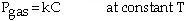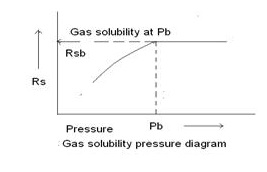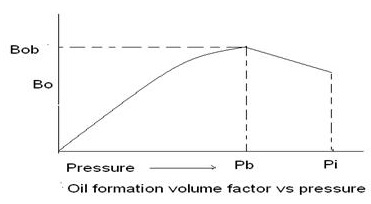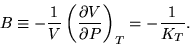# Solubility of Gases

## What is Solubility?

• Solubility is simply defined as an ability of a substance to dissolve. In the process of dissolving, the substance which is being dissolved is called a solute and the substance in which the solute is dissolved is called a solvent. A mixture of solutes and solvent is called a solution.
• Solubility is the well known property of a solid, liquid, gas or gaseous chemical substance called solute to dissolve in a solid, liquid, or gaseous solvent to form a homogeneous solution of the solute in the solvent. The solubility of a any substance fundamentally depends on the used solvent as well as on temperature and pressure.

### For Example:

In this example, you can easily understand.
Suppose, when we insert sugar into water it will dissolve. In this process:

• Sugar is the solute.
• Water is the solvent.

Generally, solubilities of solids in liquids increase with temperature and those of gases decrease with temperature and increase with pressure. A solution in which no more solute can be dissolved at a given temperature and pressure is said to be saturated (saturation).

There are various factors affecting solubility namely; temperature, pressure, polarity, molecular size and stirring increases the speed of dissolving.

## Solubility of gases/Gas solubility in liquids

The solubility of a gas in a liquid depends on temperature, the partial pressure of the gas over the liquid, the nature of the solvent and the nature of the gas. The most common solvent is water. Gas solubility is always limited by the equilibrium between the gas and a saturated solution of the gas. The dissolved gas will always follow Henry's law.

### What is Henry's Law?

The law, which was first formulated in 1803 by the English physician and chemist William Henry, holds only for dilute solutions and low gas pressures. According to Henry's law:- The solubility of a gas in a solvent is directly proportional to the partial pressure of that gas above the solvent. This relationship is written as:Where k is a temperature-dependent constant, p is the partial pressure (atm) of the solute in the gas above the solution, and c is the concentration of the dissolved gas in the liquid (mol/L). The units on {"k"} depend on the units used for concentration and pressure.

## Gas Solubility

The gas solubility Rs is defined as the number of standard cubic feet of gas which will dissolve in one stock-tank barrel of crude oil at certain pressure and temperature. The solubility of a natural gas in a crude oil is a strong function of the pressure, temperature, API gravity, and gas gravity. For a particular gas and crude oil to exist at a constant temperature, the solubility increases with pressure until the saturation pressure is reached.

At the saturation pressure (bubble point pressure) all the available gases are dissolved in the oil and the gas solubility reaches its maximum value. Rather than measuring the amount of gas that will dissolve in a given stock-tank crude oil as the pressure is increased, it is customary to determine the amount of gas that will come out of a sample of reservoir crude oil as pressure decreases.A typical gas solubility curve, as a function of pressure for an under saturated crude oil, is shown in above. As the pressure is reduced from the initial reservoir pressure pi, to the bubble point pressure pb, no gas evolves from the oil and consequently the gas solubility remains constant at its maximum value of Rsb . Below the bubble point pressure, the solution gas is liberated and the value of Rs decreases with pressure.

### What Is Bubble Point Pressure?

The bubble point pressure is defined as the pressure at which the first gas bubble appears while decreasing the pressure on the fluid sample. To determine when the first gas bubble appears is a very challenging and experimental task. e.g. The bubble point pressure pb of a hydrocarbon system is defined as the highest pressure at which a bubble of gas is first liberated from the oil. This important property can be measured experimentally for a crude oil system by conducting a constant-composition expansion test.

In the absence of the experimentally measured bubble point pressure, it is necessary for the engineer to make an estimate of this crude oil property from the readily available measured producing parameters. Several graphical and mathematical correlations for determining pb have been proposed during the last four decades. These correlations are essentially based on the assumption that the bubble point pressure is a strong function of Gas solubility Rs, Gas gravity gg, Oil gravity API , and temperature T. Thus pb is function of following factors:

pb = f (RS, gg, API, T)

### Oil formation volume factor

The oil formation volume factor (Bo) , is defined as the ratio of the volume of oil (plus the gas in solution) at the prevailing reservoir temperature and pressure to the volume of oil at standard conditions. Bo is always greater than or equal to unity (1). The oil formation volume factor can be expressed mathematically as:

Bo = (V o) p, T / (Vo) sc

Where

Bo = Oil formation volume factor, bbl/STB

(Vo)p,T = Volume of oil under reservoir pressure p and temperature T, bbl

(Vo)sc = Volume of oil is measured under standard conditions, STB

A typical oil formation factor curve, as a function of pressure for an under saturated crude oil (pi > pb), is shown in Figure. As the pressure is reduced below the initial reservoir pressure pi, the oil volume increases due to the oil expansion. This behavior results in an increase in the oil formation volume factor and will continue until the bubble-point pressure is reached. At pb, the oil reaches its maximum expansion and consequently attains a maximum value of Bob for the oil formation volume factor. As the pressure is reduced below pb, volume of the oil and Bo are decreased as the solution gas is liberated. When the pressure is reduced to atmospheric pressure and the temperature to 60°F, the value of Bo is equal to one.### Isothermal compressibility coefficient of crude oil

Isothermal compressibility coefficients are required in solving many reservoir engineering problems, including transient fluid flow problems, and they are also required in the determination of the physical properties of the under saturated crude oil.

By definition, the isothermal compressibility of a substance is defined mathematically by the following expression: For a crude oil system, the isothermal compressibility coefficient of the oil phase B is defined for pressures above the bubble point by one of the following equivalent expressions:where V is volume of the fluid and K T is isothermal bulk modulus, respectively.

### Crude oil viscosity

Crude oil viscosity is an important physical property that controls and influences the flow of oil through porous media and pipes. The viscosity, in general, is defined as the internal resistance of the fluid to flow. Oil viscosity is a strong function of many thermodynamic and physical properties such as pressure, temperature, solution gas-oil ratio, bubble point pressure, gas gravity and oil gravity. Usually oil viscosity is determined by laboratory measurements at reservoir temperature.